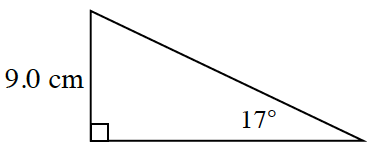Home > INT2 > Chapter 4 > Lesson 4.1.1 > Problem4-9

4-9.Calculate the perimeter of the shape at right. Clearly show all your steps. Homework Help ✎

Use slope ratios to solve for the length of the missing leg.

Use the Pythagorean Theorem to calculate the length of the hypotenuse.

Calculate the perimeter by adding up all the sides.